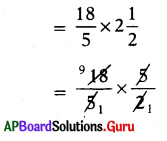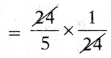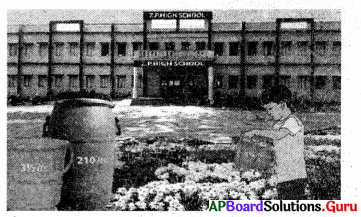# AP Board 7th Class Maths Solutions Chapter 2 Fractions, Decimals and Rational Numbers Ex 2.1

SCERT AP 7th Class Maths Solutions Pdf Chapter 2 Fractions, Decimals and Rational Numbers Ex 2.1 Textbook Exercise Questions and Answers.

## AP State Syllabus 7th Class Maths Solutions 2nd Lesson Fractions, Decimals and Rational Numbers Exercise 2.1

Question 1.
In Jagananna Gorumudda (MDM) scheme each student got $$\frac{3}{20}$$ kg. rice per day, find the weight of the rice required for 60 students in a class per day.
Given quantity of rice each student get per day = $$\frac{3}{20}$$ kg.
Quantity of rice 60 students get per day
= $$\frac{3}{20} \times \frac{60}{1}$$
= $$\frac{3 \times 60}{20 \times 1}$$
= $$\frac{180}{20}$$ = 9

∴ Quantity of rice required for 60 students per day = 9 kg.

Question 2.
What is a perimeter of an equilateral triangle, if each*side of a triangle is 5$$\frac{3}{10}$$cm?
Let the side of an equilateral triangle be x cm.
Perimeter of an equilateral triangle = 3x.
= 3 × 5 $${30}{10}$$
= $$\frac{3}{1} \times \frac{53}{10}$$
= $$\frac{3 \times 53}{1 \times 10}$$

∴ Perimeter of triangle = $$\frac{159}{10}$$ or 15$$\frac{9}{10}$$ cmQuestion 3.
Surya can walk $$\frac{18}{5}$$kilo meters in an hour. How much distance can he walk in 2$$\frac{1}{2}$$ hours ?
Given, distance walked by Surya in 1 hour = $$\frac{18}{5}$$km.
Distance walked by Surya in 2$$\frac{1}{2}$$hours∴ Distance walked in 2$$\frac{1}{2}$$hours
= $$\frac{9}{1}$$ = 9 km

Question 4.
If the length and breadth of a rectan-gular garden are $$\frac{27}{2}$$m and $$\frac{15}{2}$$m respectively, then find the area of the garden.
Given, the length of a garden = $$\frac{27}{2}$$m.
breadth of a garden = $$\frac{15}{2}$$m.
Area of rectangular garden = length × breadth
= $$\frac{27}{2} \times \frac{15}{2}$$
= $$\frac{27 \times 15}{2 \times 2}$$

∴ Area of the garden = $$\frac{405}{4}$$ or 101$$\frac{1}{4}$$

Question 5.
Gopal bought 3$$\frac{1}{2}$$kg. of potatoes in the market. If he paid ?84, then find the cost of 1 kg. of potatoes.
Given, cost of 3$$\frac{1}{2}$$ kgs of potatoes = ₹ 84
Cost of 1 kg of potatoes = 84 ÷ 3$$\frac{1}{2}$$
= 84 ÷ $$\frac{7}{2}$$
= $$\frac{84}{1} \times \frac{2}{7}$$

∴ Cost of one kg of potatoes = $$\frac{168}{7}$$
= ₹ 24

Question 6.
A car travelled 225 km. in 4 $$\frac{1}{2}$$ hours with uniform speed. Find the distance travelled in 1 hour.
Given, distance travelled in 4$$\frac{1}{2}$$ hours = 225 km
Distance travelled in 1 hour
= 225 ÷ 4$$\frac{1}{2}$$
= 225 ÷ $$\frac{9}{2}$$
= $$\frac{225}{1} \times \frac{2}{9}$$
= $$\frac{225 \times 2}{1 \times 9}$$

∴ Distance travelled by car in 1 hour
= $$\frac{450}{2}$$ = 50 km

Question 7.
If 24 students share 4$$\frac{4}{5}$$kg. of cake, then how much cake does each one get?
Given, quantity of cake shared by 24 students = 4$$\frac{4}{5}$$kg

Quantity of cake each one got
= 4$$\frac{4}{5}$$ ÷ 24 .
= $$\frac{24}{5} \div \frac{24}{1}$$∴ Quantity of cake each one got = $$\frac{1}{5}$$kg (or) 0.2 kg (or) 200 gm.Question 8.
A drum contains 210 l of water. How many times does the boy get the water for watering die plants with 3$$\frac{1}{2}$$ l of full bucket from the drum ?Capacity of bucket = 3$$\frac{1}{2}$$ l
Number of buckets of water the boy get = 210 ÷ 3$$\frac{1}{2}$$
= 210 ÷ $$\frac{7}{2}$$
= $$\frac{210}{1} \times \frac{2}{7}$$
= $$\frac{420}{7}$$ = 60